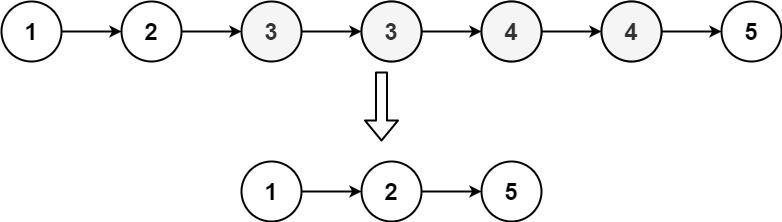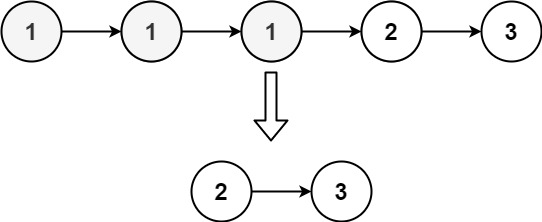# 82. Remove Duplicates from Sorted List II | LeetCode Solution

## 82. Remove Duplicates from Sorted List II | LeetCode Solution

Given the `head` of a sorted linked list, delete all nodes that have duplicate numbers, leaving only distinct numbers from the original list. Return the linked list sorted as well.

Example 1:```Input: head = [1,2,3,3,4,4,5]
Output: [1,2,5]
```

Example 2:```Input: head = [1,1,1,2,3]
Output: [2,3]
```

Constraints:

• The number of nodes in the list is in the range `[0, 300]`.
• `-100 <= Node.val <= 100`
• The list is guaranteed to be sorted in ascending order.

/**
* struct ListNode {
*     int val;
*     ListNode *next;
*     ListNode() : val(0), next(nullptr) {}
*     ListNode(int x) : val(x), next(nullptr) {}
*     ListNode(int x, ListNode *next) : val(x), next(next) {}
* };
*/
class Solution {
public:
unordered_map<int,int>m;
while(temp!=NULL){
m[temp->val]++;
temp=temp->next;
}
ListNode* ans=NULL;
bool found=false;
while(temp!=NULL){
auto it=m.find(temp->val);
if(it->second==1){
prev=temp;
if(!found){
ans=temp;
found=true;
}
}
else{
if(temp->next!=NULL){
prev->next=temp->next;
//  cout<<temp->data<<endl;
}
else{
prev->next=NULL;
}
}
temp=temp->next;

}
return ans;
}
};